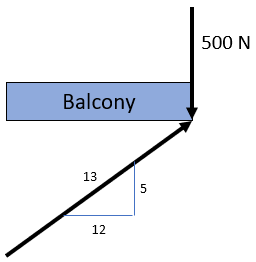## On a Balcony

Consider a person applies a downward 500 N force by standing on a balcony's edge, which is only supported by one diagonal beam. Determine the force in the support based on the diagram below. Assume the balcony is weightless.Hint
$$Force=mass\times acceleration$$$Hint 2 The problem is asking to solve for the y-component of the diagram's sum of forces: $$\sum F_y=0$$$
Since the person standing on the balcony only exerts force in the vertical/y-direction, we only need to determine the support's y-component:
$$\sum F_y=0: -500N+\frac{5}{13}F_{support}=0$$$$$F_{support}=\frac{500N\cdot 13}{5}=1300\:N$$$
1300 N